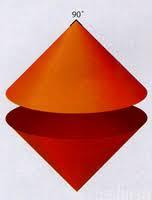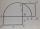# Conical area

A right angled triangle has sides a=12 and b=19 in right angle. The hypotenuse is c. If the triangle rotates on the c side as axis, find the volume and surface area of conical area created by this rotation.

Result

V =  2422.438
S =  988.1

#### Solution:

$a = 12 \ \\ b = 19 \ \\ c = \sqrt{ a^2+b^2 } = \sqrt{ 12^2+19^2 } = \sqrt{ 505 } \doteq 22.4722 \ \\ c_{ 1 } = a^2/c = 12^2/22.4722 \doteq 6.4079 \ \\ c_{ 2 } = b^2/c = 19^2/22.4722 \doteq 16.0643 \ \\ h = \sqrt{ c_{ 1 } \cdot \ c_{ 2 } } = \sqrt{ 6.4079 \cdot \ 16.0643 } \doteq 10.1459 \ \\ S_{ 1 } = \pi \cdot \ h^2 = 3.1416 \cdot \ 10.1459^2 \doteq 323.3912 \ \\ V = (c_{ 1 }+c_{ 2 }) \cdot \ S_{ 1 }/3 = (6.4079+16.0643) \cdot \ 323.3912/3 \doteq 2422.4377 = 2422.438$
$S = \pi \cdot \ h \cdot \ (a+b) = 3.1416 \cdot \ 10.1459 \cdot \ (12+19) \doteq 988.0996 = 988.1$Our examples were largely sent or created by pupils and students themselves. Therefore, we would be pleased if you could send us any errors you found, spelling mistakes, or rephasing the example. Thank you!

Leave us a comment of this math problem and its solution (i.e. if it is still somewhat unclear...):Be the first to comment!Tips to related online calculators
Looking for a statistical calculator?
Tip: Our volume units converter will help you with the conversion of volume units.

## Next similar math problems:

1. Squares above sidesTwo squares are constructed on two sides of the ABC triangle. The square area above the BC side is 25 cm2. The height vc to the side AB is 3 cm long. The heel P of height vc divides the AB side in a 2: 1 ratio. The AC side is longer than the BC side. Calc
2. Sides of the triangleCalculate triangle sides where its area is S = 84 cm2 and a = x, b = x + 1, xc = x + 2
3. Right isosceles triangleRight isosceles triangle has an altitude x drawn from the right angle to the hypotenuse dividing it into 2 equal segments. The length of one segment is 5 cm. What is the area of the triangle?
4. Right 24Right isosceles triangle has an altitude x drawn from the right angle to the hypotenuse dividing it into 2 unequal segments. The length of one segment is 5 cm. What is the area of the triangle? Thank you.
5. Goat and circlesWhat is the radius of a circle centered on the other circle and the intersection of the two circles is equal to half the area of the first circle? This task is the mathematical expression of the role of agriculture. The farmer has circular land on which
6. Isosceles triangle 9Given an isosceles triangle ABC where AB= AC. The perimeter is 64cm and altitude is 24cm. Find the area of the isosceles triangle
7. Same areaThere is a given triangle. Construct a square of the same area.
8. Hypotenuse - RTA triangle has a hypotenuse of 55 and an altitude to the hypotenuse of 33. What is the area of the triangle?
9. Medians in right triangleIt is given a right triangle, angle C is 90 degrees. I know it medians t1 = 8 cm and median t2 = 12 cm. .. How to calculate the length of the sides?
10. Triangle ABCIn a triangle ABC with the side BC of length 2 cm The middle point of AB. Points L and M split AC side into three equal lines. KLM is isosceles triangle with a right angle at the point K. Determine the lengths of the sides AB, AC triangle ABC.
11. RT sidesFind the sides of a rectangular triangle if legs a + b = 17cm and the radius of the written circle ρ = 2cm.
12. Euclid theoremsCalculate the sides of a right triangle if leg a = 6 cm and a section of the hypotenuse, which is located adjacent the second leg b is 5cm.
13. Euclid 5Calculate the length of remain sides of a right triangle ABC if a = 7 cm and height vc = 5 cm.
14. Triangle KLMIn the rectangular triangle KLM, where is hypotenuse m (sketch it!) find the length of the leg k and the height of triangle h if hypotenuse's segments are known mk = 5cm and ml = 15cm
15. CirclesIn the circle with a radius 7.5 cm are constructed two parallel chord whose lengths are 9 cm and 12 cm. Calculate the distance of these chords (if there are two possible solutions write both).
16. Euclidean distanceCalculate the Euclidean distance between shops A, B and C, where: A 45 0.05 B 60 0.05 C 52 0.09 Wherein the first figure is the weight in grams of bread and second figure is price in USD.
17. Isosceles IVIn an isosceles triangle ABC is |AC| = |BC| = 13 and |AB| = 10. Calculate the radius of the inscribed (r) and described (R) circle.